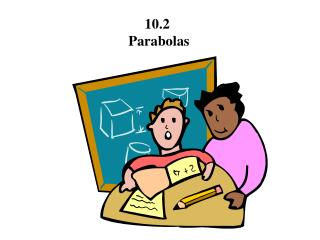DownloadDownload Presentation10.2 Parabolas

# 10.2 Parabolas

Télécharger la présentation## 10.2 Parabolas

- - - - - - - - - - - - - - - - - - - - - - - - - - - E N D - - - - - - - - - - - - - - - - - - - - - - - - - - -
##### Presentation Transcript

1. 10.2 Parabolas

2. 10.2 Parabolas A parabola is the set of all points (x,y) that are equidistant from a fixed line (directrix) and a fixed point (focus) not on the line. Focus (h, k + p) Vertex (h,k) Directrix y = k - p

3. Standard Equation of a Parabola (x - h)2 = 4p(y - k) Vertical axis Opens up (p is +) or down (p is -) (y - k)2 = 4p(x - h) Horizontal axis Opens right (p is +) or left (p is -)

4. Ex. Find the vertex, focus, and directrix of the parabola and sketch its graph. y2 + 4y + 8x - 12 = 0 Now complete the square. y2 + 4y = -8x + 12 y2 + 4y + 4 = -8x + 12 + 4 (y + 2)2 = -8x + 16 Write down the vertex and plot it. Then find p. (y + 2)2 = -8(x - 2) 4p = -8 p = -2 What does the negative p mean? left

5. Directrix x = 4 V(2,-2) F(0,-2) Homework: 5 - 29 odd

6. Ex. Find the standard form of the equation of the parabola with vertex (2,1) and focus (2,4). First, plot the two points. Which equation will we be using? Vert. or Horz. axis Right, since the axis is vertical, we will be using (x - h)2 = 4p(y - k) What is p? p = 3 Now write down the equation. (x - 2)2 = 12(y - 1)